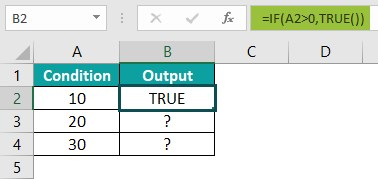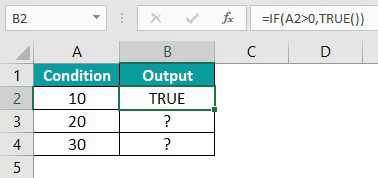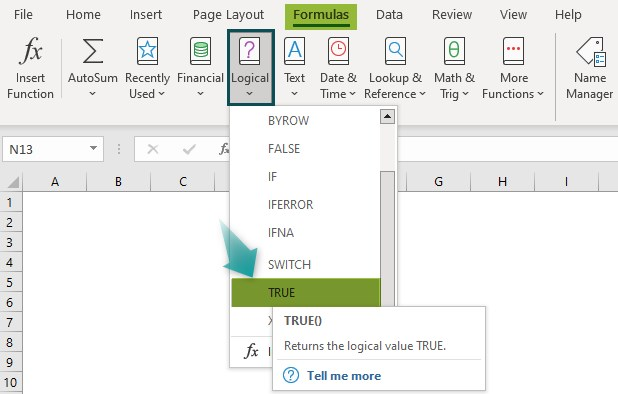# TRUE Excel FunctionArticle byExcelMojo Team## What Is TRUE Function In Excel?

The TRUE Excel function always returns only one of the BOOLEAN values, i.e., “True”, as the output. It is used with conditional functions such as IF(), ERROR(), etc. to get an alternate or a conditional output. The TRUE Function in Excel is an inbuilt logical function in Excel, so we can insert the formula from the “Function Library” or enter it directly in the worksheet.

For example, we will apply the TRUE function to calculate the logical condition. Enter the formula =TRUE in cell A2 and =TRUE() in cell A3, and press “Enter.

The results are returned as ‘TRUE’, as shown above. Column B is for our reference.

###### Key Takeaways
• The TRUE Excel Function returns the value “TRUE” as the output.
• The function helps us to understand whether a given condition is true or not when we use it within the formulas or other Excel functions such as IF(), ERROR(), etc.
• Excel has a default value for “TRUE” as 1, and for “FALSE” as 0. So, we perform arithmetic or numeric calculations for the function with the default values.
For ex, =True+11 is 1+11 = 1.
• The TRUE function is used with or without a bracket. The result will be the same, as the function doesn’t accept any arguments.

### TRUE() Excel Formula

The syntax of the TRUE Excel formula is,

The function takes no argument and always returns the logical value, i.e., TRUE.

### How To Use TRUE Excel function?

We can use the TRUE Excel function in 2 methods, namely,

1. Access from the Excel ribbon.
2. Enter in the worksheet manually.

#### Method #1 – Access from the Excel ribbon

First, choose an empty cell – select the “Formulas” tab – go to the “Function Library” group – click the “Logical” option drop-down – select the “TRUE” function, as shown below.

The “Function Arguments” window appears. As the function takes no argument, click “OK”, as shown below.

#### Methods #2 – Enter in the worksheet manually

1. Select an empty cell for the output.
2. Type =TRUE() or =TRUE in the selected cell. [Alternatively, type “=T” and double-click the TRUE function from the list of suggestions shown by Excel.]
3. Press the “Enter” key.

#Basic Example

We will calculate the logical condition of the given data using TRUE and the IF excel function.

In the table, the data is,

• Column A: contains Condition.
• Column B: contains Output.

The steps to find the value of the condition using TRUE Excel function are,

1. Select cell B2, enter the formula =IF(A2>0,TRUE()), and press the “Enter” key. The result is “TRUE”, as shown below.2. Drag the formula from cell B2 to B4 using the fill handle.The output is shown above. The results are as per the condition of the IF() function.

### Examples

We will understand some advanced scenarios using the TRUE Excel function examples.

#### Example #1

We will calculate the logical condition of the given data using the TRUE Excel function and the IF() function.

In the table, the data is,

• Column A: contains Value1.
• Column B: contains Value2.
• Column C: contains Output.

The steps to find the conditional output using the TRUE function are,

Step 1: Select cell C2, enter the formula =IF(A2=B2,TRUE()), and press the “Enter” key. The result is “TRUE”, as shown below.

Step 2: Enter the formulas as follows, =IF(A2>B3,TRUE()) in cell B3, =IF(A2<B3,TRUE()) in cell B4, =(10>1) in cell B4, and =(10>1) in cell B5. Press the “Enter” key every time the formula is entered.

The output is shown above. Column D is for our reference.

#### Example #2

We will calculate the logical conditions of the given data without the TRUE function by entering the given values as formulas to get either “TRUE” or “FALSE”, as per the conditions.

In the table, the data is,

• Column A: contains Condition.
• Column B: contains Output.

The procedure to find the logical values of the given conditions are,

Enter the formulas as follows, =(10=10)in cell B2, =(10>1) in cell B3, =(10<100) in cell B4, and =(10<>100) in cell B5. Press the “Enter” key every time the formula is entered.

We will get the output shown above.

#### Example #3

We will perform some arithmetic expressions with the TRUE function and calculate the logical conditions.

In the table, the data is,

• Column A: contains Condition.
• Column B: contains a Formula that explains the expressions used in the example.

The procedure to find the logical values of the given conditions are,

Enter the formulas as follows, =TRUE*10 in cell A2, =TRUE/1 in cell A3, =TRUE-1 in cell A4, and =TRUE+1 in cell A5. Press the “Enter” key every time the formula is entered.

The results are shown above. Column B is for our reference.

### Important Things To Note

• The logical expressions will automatically return TRUE and FALSE as results.
• The TRUE function was introduced in Microsoft Excel in 2007. For calculation purposes, the default value of TRUE is 1, and FALSE is 0.
• TRUE+TRUE=2
• FALSE+TRUE=1
• We also can perform arithmetic calculations with TRUE() like: TRUE() * 8  = 8
• If we want to enter TRUE, or if we want TRUE as a result in a TRUE Excel formula, just type TRUE directly in the cell. Excel will return it as the logical value TRUE as output. For example: =IF (A1<0, TRUE ()) or =IF (A1<0, TRUE)

1. What does the TRUE function do in Excel?

The TRUE Excel Function is the Boolean logical function that returns TRUE, which is equal to 1, as an output. The function is equivalent to directly using the Boolean value TRUE in a formula.

The syntax of the TRUE Function in Excel is =TRUE().

2. How does the TRUE function work in Excel?

We can work with the TRUE function as follows:

1. Select an empty cell for the output.
2. Type =TRUE() or =TRUE in the selected cell. [Alternatively, type “=T” and double-click the TRUE function from the list of suggestions shown by Excel.]
3. Press the “Enter” key.

3. Where is the TRUE Function in Excel?

We can insert the Excel TRUE Function as shown below:

Choose the empty cell which will contain the result.
Go to the “Formulas” tab and click it.
Select the “Logical” option from the drop-down menu.